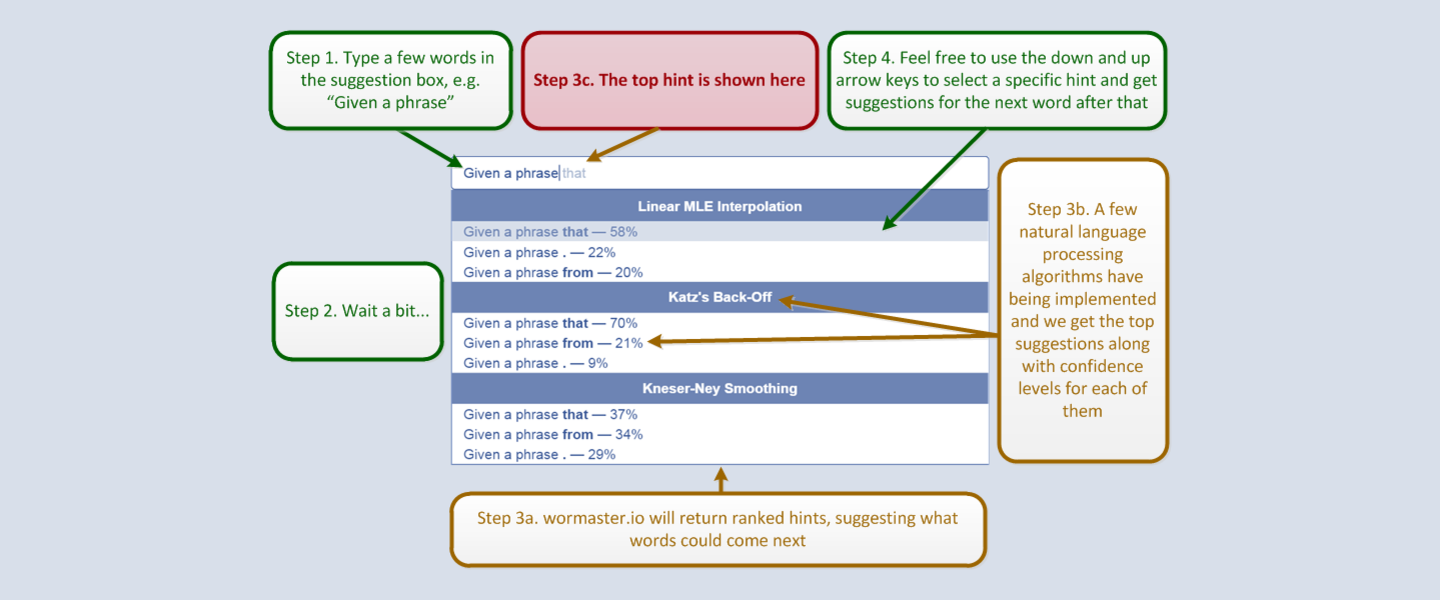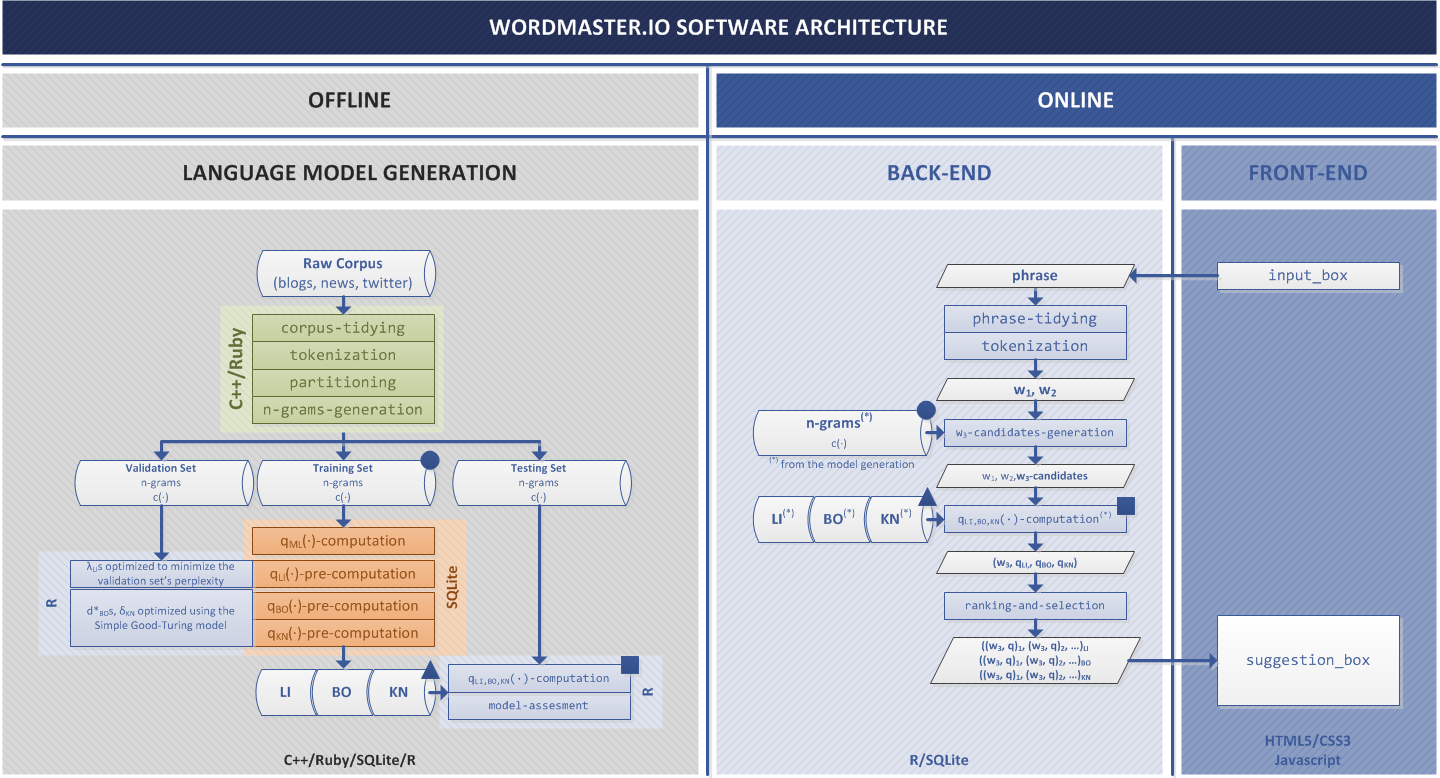• Ivan Corneillet
• April 2015

### wordmaster.io is easy and intuitive to use thanks to its familiar Google-like "suggestion" box design### Under the hood: This next-word prediction business is pretty cool, hein? How does it work? Natural Language Processing (NLP) to the rescue...

$$n$$-gram Language Models

We implemented three $$n$$-gram language models that use different intuitions to deal with a key observation on data sparsity, namely that a large number of $$n$$-grams occur with such low frequency that they haven't been observed them in the training dataset.

• Linear MLE Interpolation (LI): Interpolation deals with these rare n-grams by “consulting” all $$n$$-grams models at the same time.
• Katz's Back-Off (BO): Instead off mixing all models at the same time start with the most detail model (with the highest $$n$$) and only “hierarchically” back-off to a lower order $$n$$-gram model if there is no evidence of the higher-order $$n$$-gram.
• Kneser-Ney Smoothing (KN): Words that have appeared in more contexts are more likely to appear in new contexts. E.g., Likelihood of “Angeles” is high and a normal back-off will likely pick it in any context; yet “Angeles” occurs with only a single context (only occurs after “Los”).

Next-Word Prediction

With these language models in place, we then predict the next words as follow:

• (1) Compile the list of words $$w$$ such that: $$w: \begin{cases} c(w_{1}, w_{2}, w) > 0 \\ \text{; (select } w \text{ so that the 3-gram } w_{1}, w_{2}, w \text{ is in our model)} \\ c(w_{1}, w_{2}, w) = 0 \text{ and } c(w_{2}, w) > 0 \\ \text{; (select } w \text{ so that the 2-gram } w_{2}, w \text{ is in our model but the 3-gram } w_{1}, w_{2}, w \text{ is not)} \end{cases}$$
• (2) If the list is empty, return the most frequent 1-gram “the”.
• Otherwise: (3) evaluate $$q(w | w_{1}, w_{2})$$ for each word $$w$$ in the list,
• (4) sort the list by decreasing $$q$$,
• and (5) return the top $$5$$ results.

(Note: We used $$1$$-, $$2$$-, and $$3$$-grams)

### Diving deeper: Multiple variants of the language models we implemented exist. Here are the formulas we used.

$$Variables$$ $$3-grams$$ $$2-grams$$ $$1-grams$$
$$q_{ML}(\cdot)$$(1,2) $$q_{ML}(w_{3} | w_{2}, w_{1}) = \frac{c(w_{1}, w_{2}, w_{3})}{c(w_{1}, w_{2})}$$ $$q_{ML}(w_{2} | w_{1}) = \frac{c(w_{1}, w_{2})}{c(w_{1})}$$ $$q_{ML}(w_{1}) = \frac{c(w_{1})}{c(\cdot)}$$
$$q_{LI}(\cdot)$$(1,2) $$q_{LI}(w_{3} | w_{2}, w_{1}) = \lambda^{3}_{3} \cdot q_{ML}(w_{3} | w_{2}, w_{1})$$ $$+ \lambda^{3}_{2} \cdot q_{ML}(w_{3} | w_{2})$$ $$+ \lambda^{3}_{1} \cdot q_{ML}(w_{3})$$ $$q_{LI}(w_{2} | w_{1}) = \lambda^{2}_{2} \cdot q_{ML}(w_{2} | w_{1}) + \lambda^{2}_{1} \cdot q_{ML}(w_{2})$$ $$q_{LI}(w_{1}) = q_{ML}(w_{1})$$
$$d^{*}(\cdot)$$(3) $$d^{*}(w_{1}, w_{2}, w_{3}) = \left (1 + \frac{1}{c(w_{1}, w_{2}, w_{3})} \right ) ^ {1+b_{3}}$$ $$d^{*}(w_{1}, w_{2}) = \left (1 + \frac{1}{c(w_{1}, w_{2})} \right ) ^ {1+b_{2}}$$ $$d^{*}(w_{1}) = \left (1 + \frac{1}{c(w_{1})} \right ) ^ {1+b_{1}}$$
$$q_{BO}(\cdot)$$(1,2,4) $$q_{BO}(w_{3} | w_{2}, w_{1}) = \begin{cases} d^{*}(w_{1}, w_{2}, w_{3}) \cdot q_{ML}(w_{3} | w_{1}, w_{2}) \\ \text{ if } c(w_{1}, w_{2}, w_{3}) > 0 \\ \alpha(w_{1}, w_{2}) \cdot q_{BO}(w_{3} | w_{2}) \\ \text{ otherwise} \end{cases}$$ $$\alpha(w_{1}, w_{2}) = \frac{\beta(w_{1}, w_{2})}{\sum_{w_{i}: c(w_{1}, w_{2}, w_{i}) \leq k} q_{BO}(w_{2}, w_{i})}$$ $$\beta(w_{1}, w_{2}) = 1 - \sum_{w_{i}: c(w_{1}, w_{2}, w_{i}) > 0} d^{*}(w_{1}, w_{2}, w_{i}) \cdot q_{ML}(w_{1}, w_{2}, w_{i})$$ $$q_{BO}(w_{2} | w_{1}) = \begin{cases} d^{*}(w_{1}, w_{2}) \cdot q_{ML}(w_{2} | w_{1}) \\ \text{ if } c(w_{1}, w_{2}) > 0 \\ \alpha(w_{1}) \cdot q_{BO}(w_{2}) \\ \text{ otherwise} \end{cases}$$ $$\alpha(w_{1}) = \frac{\beta(w_{1})}{\sum_{w_{i}: c(w_{1}, w_{i}) \leq k} q_{BO}(w_{i})}$$ $$\beta(w_{1}) = 1 - \sum_{w_{i}: c(w_{1}, w_{i}) > 0} d^{*}(w_{1}, w_{i}) \cdot q_{ML}(w_{1}, w_{i})$$ $$q_{BO}(w_{1}) = d^{*}(w_{1}) \cdot q_{ML}(w_{1})$$
$$q_{KN}(\cdot)$$(2,5) $$q_{KN}(w_{3} | w_{1}, w_{2}) = \frac{max(c(w_{1}, w_{2}, w_{3}) - \delta, 0)}{\sum_{w_{i}} c(w_{1}, w_{2}, w_{i})}$$ $$+ \lambda(w_{1}, w_{2}) \cdot q_{KN}(w_{3} | w_{2})$$ $$\lambda(w_{1}, w_{2}) = \frac{\delta}{\sum_{w_{i}} c(w_{1}, w_{2}, w_{i})} \cdot \left | w_{i} \right |_{c(w_{1}, w_{2}, w_{i}) > 0}$$ $$q_{KN}(w_{2} | w_{1}) = \frac{max(c(w_{1}, w_{2}) - \delta, 0)}{\sum_{w_{i}} c(w_{1}, w_{i})}$$ $$+ \lambda(w_{1}) \cdot q_{KN}(w_{2})$$ $$\lambda(w_{1}) = \frac{\delta}{\sum_{w_{i}} c(w_{1}, w_{i})} \cdot \left | w_{i} \right |_{c(w_{1}, w_{i}) > 0}$$ $$q_{KN}(w_{1}) = \frac{\left | w_{i} \right |_{c(w_{i}, w_{1}) > 0}}{\left | (w_{i}, w_{j}) \right |_{c(w_{i}, w_{j}) > 0}}$$

Please note that we used the Good–Turing Smoothing method to compute the discountings used by Katz's Back-Off and Kneser-Ney Smoothing (Good–Turing isn't a model by itself).

### wordmaster.io follows a three-tier architecture. What you see in your browser is literally the top of the iceberg...

In the offline language model phase, we pre-computed all $$\alpha$$'s, $$\beta$$'s, $$\delta$$'s, $$d^{*}$$'s, and $$q^{*}$$'s for our (observed) $$n$$-grams ($$1 \leq n \leq 3$$). These building blocks were then stored in a SQLite database.

These pre-computed blocks stored a SQL database leave minimal work to the back-end (queries are simple with minimal joins) and further minimize the memory footprint (The model stays on disk and little data is loaded into main memory).

For more information on the data clean-up and the $$n$$-grams generation, please refer to our early report.